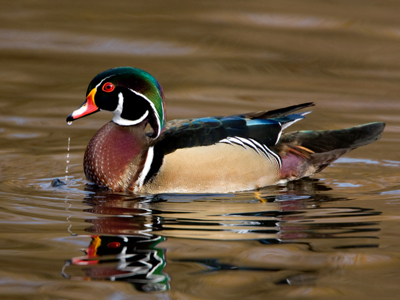Do you think the number 2 resembles a duck?

# 11 Times Table

Learning the times tables is a vital tool when multiplying numbers. By the end of Year 2 children are often fluent in their times tables up to 10. When they enter into KS2 in Year 3 they will also be taught some higher times tables in their Maths and Numeracy lessons. By the end of KS2 they will be familiar with all of the times tables from one to twelve. This quiz will test their knowledge of the 11 times table.

Learning the 11 times table is all about multiplying numbers by 11. The 11 times table can be a little tricky, especially when multiplying the larger numbers. Keep in mind the much easier 10 times table and that should help.

If you've been learning your times tables see if you can get full marks in this 11 times table quiz!

1.
8 x 11 =
81
88
89
98
2.
3 x 11 =
31
33
35
37
3 x 11 is the same as writing 11 + 11 + 11
3.
11 x 11 =
118
119
120
121
For these double figures, it's worth working out 10 x 11 and then simply adding another 11 onto the total
4.
11 x 4 =
41
43
44
49
5.
11 x 12 =
112
132
144
157
6.
11 x 7 =
77
71
75
79
7.
5 x 11 =
51
56
59
55
For single figures, you can simply change the '11' into the same numbers as you're multiplying them by. So 11 becomes 55. Easy!
8.
11 x 9 =
91
99
101
111
A 99 is an ice-cream with a chocolate flake sticking out! Yum
9.
2 x 11 =
19
20
21
22
In bingo, 22 is sometimes called two little ducks because the numbers look a little like ducks
10.
6 x 11 =
66
65
63
61
You can find more about this topic by visiting BBC Bitesize - 11 and 12 times table

Author:  Sarah Garratty